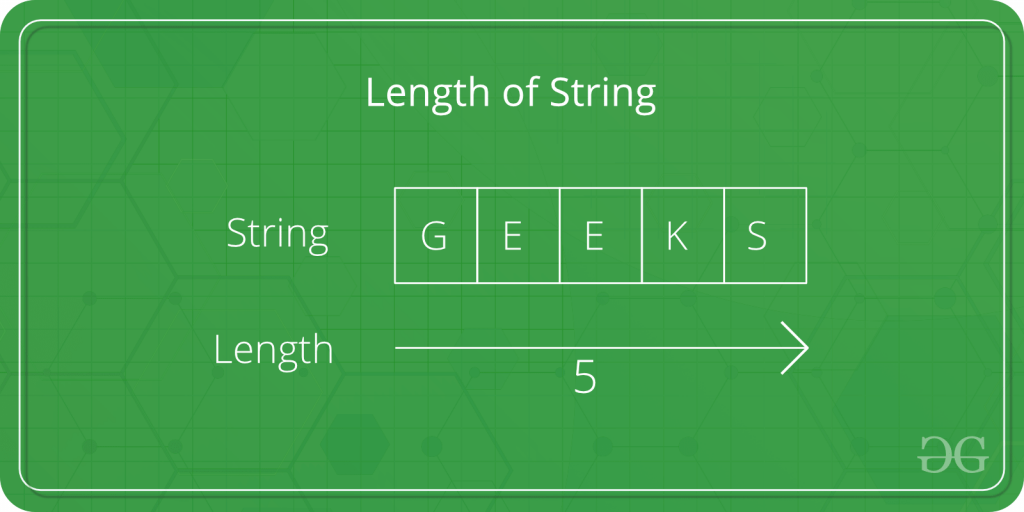Related Articles
C program to find the length of a string
• Difficulty Level : Basic
• Last Updated : 08 Oct, 2018

Given a string str. The task is to find the length of the string.Examples:

```Input: str = "Geeks"
Output: Length of Str is : 4

Input: str = "GeeksforGeeks"
Output: Length of Str is : 13
```

## Recommended: Please try your approach on {IDE} first, before moving on to the solution.

In the below program, to find the length of the string str, first the string is taken as input from the user using scanf in, and then the length of Str is calculated usingand usingmethod .

Below is the C program to find the length of the string.

Example 1: Using loop to calculate the length of string.

 `// C program to find the length of string``#include ``#include `` ` `int` `main()``{``    ``char` `Str;``    ``int` `i;`` ` `    ``printf``(``"Enter the String: "``);``    ``scanf``(``"%s"``, Str);`` ` `    ``for` `(i = 0; Str[i] != ``'\0'``; ++i);`` ` `    ``printf``(``"Length of Str is %d"``, i);`` ` `    ``return` `0;``}`
Output:
```Enter the String: Geeks
Length of Str is 5
```

Example 2: Using strlen() to find the length of the string.

 `// C program to find the length of ``// string using strlen function``#include ``#include `` ` `int` `main()``{``    ``char` `Str;``    ``int` `i;`` ` `    ``printf``(``"Enter the String: "``);``    ``scanf``(``"%s"``, Str);`` ` `    ``printf``(``"Length of Str is %ld"``, ``strlen``(Str));`` ` `    ``return` `0;``}`
Output:
```Enter the String: Geeks
Length of Str is 5
```

Want to learn from the best curated videos and practice problems, check out the C Foundation Course for Basic to Advanced C.

My Personal Notes arrow_drop_up